0
139

# Geometry – Triangles Questions for MAH – CET 2022 – Download PDF

Here you can download CMAT 2022 – important MAH – CET Triangles Questions PDF by Cracku. Very Important MAH – CET 2022 and These questions will help your MAH – CET preparation. So kindly download the PDF for reference and do more practice.

Question 1: Consider the two similar triangles ABC and DEF. Which of the following is correct about the ratio of the area of the triangle ABC and DEF?

a) $(\frac{AB}{DE})=(\frac{EF}{BC})=(\frac{AC}{DF})$

b) $(\frac{AB}{DE})^2=(\frac{BC}{EF})^2=(\frac{AC}{DF})^2$

c) $(\frac{AB}{DE})=(\frac{BC}{EF})=(\frac{DF}{AC})$

d) None of these

Question 2: Consider the two equiangular triangles ABC and DEF having medians as AL and DM respectively as shown in figure below.

a) $\frac{BC}{EF}=\frac{DM}{AL}$

b) $\frac{BC}{EF}=\frac{AL}{DM}$

c) $\frac{EF}{BC}=\frac{AL}{DM}$

d) All options are Correct

Question 3: Which of the following options is/are CORRECT about the similarity of the two triangles?

a) The corresponding sides are proportional to each other.

b) The corresponding angles are equal.

c) The corresponding sides may or may not be equal to each other.

d) All option are correct.

Question 4: In the given figure. $ABCDEF$ is a regular hexagon whose side is 6 cm. $APF, QAB, DCR$ and $DES$ are equilateral triangles. What is the area (in $cm^2$) of the shaded region?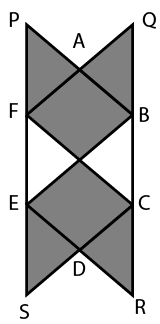a) $24\surd3$

b) $18\surd3$

c) $72\surd3$

d) $36\surd3$

Question 5: $PQRS$ is a square whose side is $20 cm$. By joining opposite vertices of $PQRS$ are get four triangles. What is the sum of the perimeters of the four triangles?

a) $40\surd2$

b) $80\surd2 + 80$

c) $40\surd2 + 40$

d) $40\surd2 + 80$

Take Free MAH-CET mock tests here

Question 6: The ratio of the areas of two triangles is 1 : 2 and the ratio of their bases is 3 : 4. What will be the ratio of their height?

a) 1 : 3

b) 4 : 3

c) 2 : 1

d) 2 : 3

Question 7: The ratio of the area of two triangles is 2 : 3 and ratio of their height is 3 : 2. The ratio of their bases is:

a) 3:2

b) 4:9

c) 9:4

d) 2:3

Question 8: The ratio of the areas of two triangles ABC and PQR is 3 : 5 and the ratio of their heights is 5 : 3. The ratio of the bases of triangle ABC to that of triangle PQR is:

a) 25 : 9

b) 9 : 25

c) 2 : 1

d) 1 : 1

Question 9: The ratio of the areas of two triangles ABC and PQR is 4: 5 and the ratio of their heights is 5 : 3. The ratio of the bases of triangle ABC to that of triangle PQR is:

a) 25 : 12

b) 12 : 25

c) 11 : 15

d) 15 : 11

Question 10: There is a polygon of 11 sides. How many triangles can be drawn by only using the vertices of the polygon?

a) 180

b) 150

c) 165

d) 175

Question 11: Equilateral triangles are drawn on the hypotenuse and one of the perpendicular sides of a right-angled isosceles triangles. Their areas are H and A respectively. $\frac{A}{H}$ is equal to:

a) $\frac{1}{4}$

b) $\frac{1}{\sqrt{2}}$

c) $\frac{1}{\sqrt{2}}$

d) $\frac{1}{2}$

Question 12: Give that the ratio of altitudes of two triangles is 4 : 5, ratio of their areas is 3: 2. The ratio of their corresponding bases is

a) 8:15

b) 5:8

c) 15:8

d) 8:5

Question 13: The sides of an isosceles triangles are 10 cm, 10 cm and 12 cm. What is the area of the triangle?

a) 60 $cm^2$

b) 48 $cm^2$

c) 40 $cm^2$

d) 44 $cm^2$

Question 14: The perimeters of two similar triangles ABC and PQRare 78 cm and 46.8 cm, respectively. If PQ = 11.7, then the length of AB is:

a) 19.5 cm

b) 23.4 cm

c) 24 cm

d) 20 cm

Question 15: Two equilateral triangles of side $10\sqrt{3}$ cm are joined to form a quadrilateral. What is the altitude of the quadrilateral?

a) 12 cm

b) 14 cm

c) 16 cm

d) i5 cm

It is given that $\triangle$ ABC $\sim\triangle$ DEF

=> $\frac{AB}{DE}=$ $\frac{BC}{EF}=$ $\frac{AC}{DF}$

Also, ratio of areas of two similar triangles is equal to the ratio of the square of the corresponding sides.

=> $\frac{ar(\triangle ABC)}{ar(\triangle DEF)}=$ $(\frac{AB}{DE})^2=(\frac{BC}{EF})^2=(\frac{AC}{DF})^2$

=> Ans – (B)

It is given that $\triangle$ ABC and $\triangle$ DEF are equiangular triangles, thus corresponding angles are equal.

=> $\triangle$ ABC $\sim\triangle$ DEF

=> Ratio of perimeter = $\frac{AB}{DE}=$ $\frac{BC}{EF}=$ $\frac{AC}{DF}$ ————-(i)

Since, AL and DM are medians, => Ratio of perimeter of $\triangle$ ABC to $\triangle$ DEF = $\frac{AL}{DM}$ ———–(ii)

From, equations (i) and (ii), we get :

=> $\frac{BC}{EF}=\frac{AL}{DM}$

=> Ans – (B)

If two triangles are similar, then the corresponding sides are proportional to each other. Also, the corresponding angles are equal, but the corresponding sides may or may not be equal to each other. Thus, all are correct.

=> Ans – (D)

We have :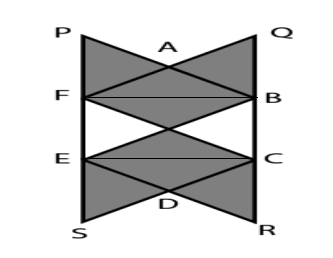We here have 6 equilateral triangles of side 6cm so we can say a complete hexagon and half area of rectangle BCFE
Now In triangle ABF
using cosine rule
we get cos A = (6^2+6^2-BF^2)/2AF AB
we get BF $6\sqrt{\ 3}$
Area of shaded region = Area of hexagon + 0.5(Area of rectangle BFCE)
we get area = $6\times\ \frac{\sqrt{\ 3}}{4}\times\ 6\times\ 6\ +\ 6\sqrt{\ 3}\times\ 6\times\ 0.5$
= $54\sqrt{\ 3}+18\sqrt{\ 3}\ =72\sqrt{\ 3}$

Side of square is 20 cm.

So, diagonal of square is 20√2 cm.

So,to calculate perimeter of triangle,we get to count each diagonal 2 times and 4 sides only one time .

So, required perimeter=4×20+4×20√2

=(80+80√2) cm.

B is correct choice.

Let the bases of the triangles be 3x and 4x units.
Heights of the triangles be a and b units respectively.
Then, Ratio of their areas = $\dfrac{1}{2}\times3x\times a : \dfrac{1}{2}\times4x\times b = 3a : 4b$
Given, $3a : 4b = 1 : 2$
=> $3a\times2 = 4b\times1 => 6a = 4b$
=> $\dfrac{a}{b} = \dfrac{4}{6} = \dfrac{2}{3}$
Therefore, Their heights will be in the ratio 2 : 3.

Let height of the two triangles be 3 and 2 units respectively.

Let bases of the two triangles be $b_1$ and $b_2$ units respectively.

=> Ratio of area of triangles = $\frac{\frac{1}{2}\times b_1\times h_1}{\frac{1}{2}\times b_2\times h_2}=\frac{2}{3}$

=> $\frac{3b_1}{2b_2}=\frac{2}{3}$

=> $\frac{b_1}{b_2}=\frac{4}{9}$

$\therefore$ Required ratio = 4 : 9

=> Ans – (B)

Let the Areas  of $\triangle ABC$ and $\triangle PQR$  are  $3x:5x$

Let the Heights  of $\triangle ABC$ and $\triangle PQR$  are  $5y:3y$  and Bases are $B_{1}$ and $B_{2}$ respectively.

Area of $\triangle ABC$ = $\frac{1}{2} \times 5y\times B_{1}$ and Area of $\triangle PQR$ = $\frac{1}{2} \times3y\times B_{2}$

$\therefore \frac{3x}{5x} = \frac{\frac{1}{2} \times 5y\times B_{1}}{\frac{1}{2} \times3y\times B_{2}}$

$\Rightarrow \frac{B_{1}}{B_{2}} = \frac{9}{25}$

$\therefore$   $B_{1}:B_{2} = 9:25$

We know that
Area = $\frac{1}{2}\times\ b\times\ h$
Now we can say
The ratio of areas of two triangles with bases b1 and b2 and heights h1 and h2 will be $\frac{\left(b1\ h1\right)}{b2\ h2}$
so we get
$\frac{4}{5}=\frac{\left(5b1\ \right)}{3b2}$
so b1:b2 =12:25

Given,

Number of sides of the polygon = 11

$=$>  Number of vertices of the polygon = 11

Number of vertices of the triangle = 3

Number of possibilities of selecting 3 from 11 = $11_{C_3}$ =$\frac{11\times10\times9}{1\times2\times3}$ = $165$

$\therefore\$Number of triangles that can be drawn by only using the vertices of the polygon of 11 sides = 165

Hence, the correct answer is Option C

Let the length of the two equal sides of right-angled isosceles triangle be x cm then hypotenuse be $x\sqrt{2} cm$

Area of equilateral triangle drawn on hypotenuse (H) = $\frac{\sqrt{3}}{4}\times a^{2}=\frac{\sqrt{3}}{4}\times (x\sqrt{2})^2$

Area of equilateral triangle drawn on side (A) = $\frac{\sqrt{3}}{4}\times a^{2}=\frac{\sqrt{3}}{4}\times x^2$

$\frac{A}{H}=\left(\frac{\sqrt{3}}{4}\times x^{2}\right)\times\frac{4}{\sqrt{3}}\times\frac{1}{(x\sqrt{2})^2} = \frac{1}{2}$

Given that ratio of altitudes of two triangles is 4:5

=> $\frac{h_{1}}{h_{2}}$=$\frac{4}{5}$

Also, Given that, ratio of areas of two triangles is 3:2

=> $\frac{\frac{1}{2} \times b_{1} \times h_{1}}{\frac{1}{2} \times b_{2} \times h_{2}}$ = $\frac{3}{2}$

=> $\frac{b_{1} \times 4}{ b_{2} \times 5}$ = $\frac{3}{2}$

=> $\frac{b_{1}}{ b_{2}}$ = $\frac{15}{8}$

Therefore, ratios of the bases is 15:8

To find the area of the isosceles triangle ABC draw a perpendicular line from A to the base of the triangle BC and name that point as D such that it will become a right angled triangle.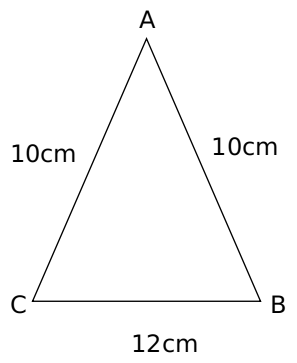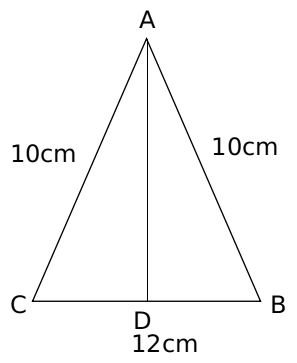Now,BD = CD =6cm.The area of the right angled triangle is given as = $\frac{1}{2}\times{b}\times{h}$,where b and h are base and height.
Height we can calculate from pythagoras theorem
$AD^{2}$ = $(AB^{2} – BD^{2})$
$\Rightarrow$ $AD^{2}$ = $(10^{2} – 6^{2})$
$\Rightarrow$ AD = 8cm.
The area of right angled now will be = $\frac{1}{2}\times{6}\times{8}$ = 24$cm^{2}$
The area of triangle ABC will be two times the area of triangle ADB = 24+24=48$cm^{2}$.
Hence option B is correct.

Triangles ABC and PQR are similar.
So,
$\frac{perimeter of ABC}{perimeter of PQR} = \frac{side of ABC}{side of PQR}$
$\frac{78}{46.8} = \frac{AB}{11.7}$
$\frac{78}{46.8} \times 11.7 = AB$
AB = 19.5 cm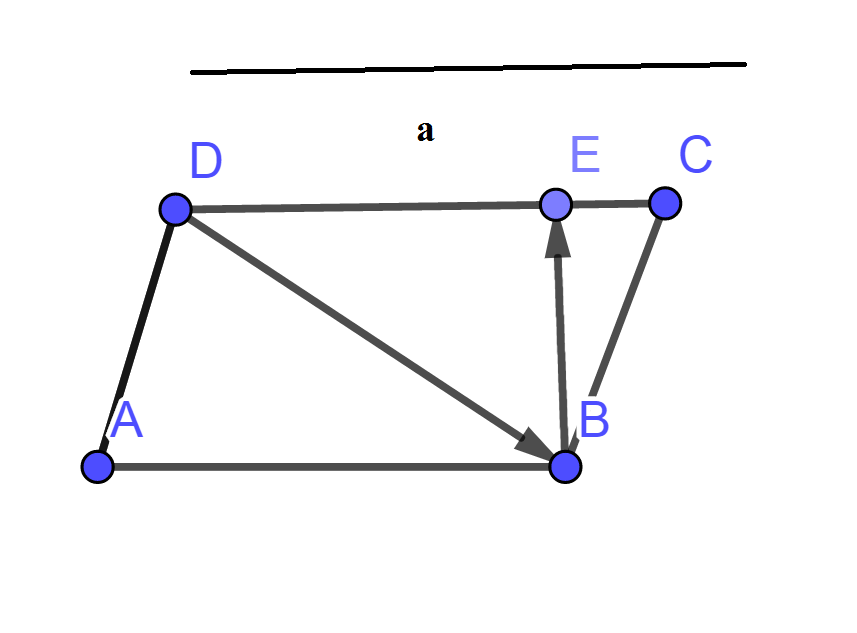Given that  $10\sqrt{3}$ cm

We know the area of equilateral triangle = $\frac{\sqrt {3}} {4} a^2$   …… Eq (1)

and on the $\triangle DCB is also given = \frac{1}{2} \times a \times h$   …… Eq (2)

then Eq(1) = Eq (2)

$\frac{\sqrt {3}} {4} a^2 = \frac{1}{2} \times a \times h$

$\Rightarrow h = \frac{\sqrt{3}} {2} a$

$\Rightarrow h = \frac{\sqrt{3}} {2} \times 10\sqrt{3}$

\$\Rightarrow h = 15 cm Ans